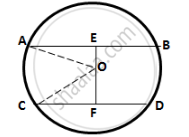Share

# Two Parallel Chords Are Drawn in a Circle of Diameter 30.0 Cm. the Length of One Chord is 24.0 Cm and the Distance Between the Two Chords is 21.0 Cm; Find the Length of Another Chord. - Mathematics

Course

#### Question

Two parallel chords are drawn in a circle of diameter 30.0 cm. The length of one chord is 24.0 cm and the distance between the two chords is 21.0 cm; find the length of another chord.

#### Solution

Since the distance between the chords is greater than the radius of the circle (15 cm), so the
chords will be on the opposite sides of the centre.Let O be the centre of the circle and AB and CD be the two parallel chords such that
AB = 24 cm.
Let length of CD be 2x cm.
Drop OE and OF perpendicular on AB and CD from the centre O.
OE ⊥ AB and OF⊥CD
∴ OE bisects AB and OF bisects CD
(Perpendicular drawn from the centre of a circle to a chord bisects it)

⇒ AE = 24/2 = 12cm; CF = (2x)/2 =x cm

In right ΔOAE,

OA2 = OE2+AE2

⇒ OE2 = OA2 - AE2 = (15)2 - (12)2 = 81

∴ OE = 9 cm
∴ OF = EF - OE = (21- 9) =12 cm

In right ΔOCF,

OC2 = OF2 + CF2

⇒ x2 = OC2 - OF2 = (15)2 -   (12)2 = 81
∴ x = 9 cm
Hence, length of chord CD = 2x = 2×9 =18 cm

Is there an error in this question or solution?